Next: 1D Newton's search Up: Annexes Previous: Notions of constrained optimization   Contents

# The secant equation

Let us define a general polynomial of degree 2: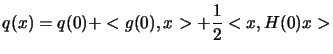(13.27)

whereare constant. From the rule for differentiating a product, it can be verified that: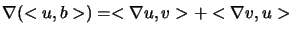ifand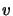depend on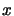. It therefore follows from 13.27 (using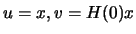) that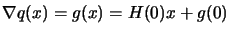(13.28)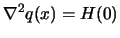A consequence of 13.28 is that if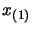and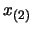are two given points and if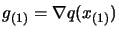and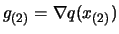(we simplify the notation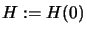), then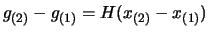(13.29)

This is called the Secant Equation''. That is the Hessian matrix maps the differences in position into differences in gradient.

Frank Vanden Berghen 2004-04-19